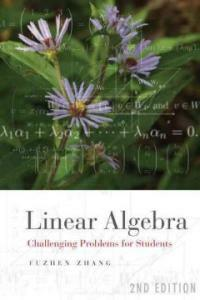> 상세정보

# 상세정보## Linear algebra : challenging problems for students 2nd ed (5회 대출)

자료유형
단행본
개인저자
Zhang, Fuzhen, 1961-.
서명 / 저자사항
Linear algebra : challenging problems for students / Fuzhen Zhang.
판사항
2nd ed.
발행사항
Baltimore, Md. :   Johns Hopkins University Press,   c2009.
형태사항
xv, 245 p. : ill. ; 23 cm.
총서사항
The Johns Hopkins studies in the mathematical sciences
ISBN
9780801891250 (hbk.) 0801891256 (hbk.) 9780801891267 (pbk.) 0801891264 (pbk.)
요약
Linear algebra is a prerequisite for students majoring in mathematics and is required of many undergraduate and first-year graduate students in statistics, engineering, and related areas. This fully updated and revised text defines the discipline's main terms, explains its key theorems, and provides over 425 example problems ranging from the elementary to some that may baffle even the most seasoned mathematicians. Vital concepts are highlighted at the beginning of each chapter and a final section contains hints for solving the problems as well as solutions to each example.Based on Fuzhen Zhang's experience teaching and researching algebra over the past two decades, Linear Algebra is the perfect examination study tool. Students in beginning and seminar-type advanced linear algebra classes and those seeking to brush up on the topic will find Zhang's plain discussions of the subject's theories refreshing and the problems diverse, interesting, and challenging.
내용주기
Vector spaces -- Determinants, inverses and rank of matrices, and systems of linear equations -- Matrix similarity, eigenvalues, eigenvectors, and linear transformations -- Special matrices -- Inner product spaces.
서지주기
Includes bibliographical references (p. 241) and index.
일반주제명
Algebras, Linear -- Problems, exercises, etc.
 000 02409camuu2200349 a 4500 001 000045630771 005 20110217093806 008 110216s2009 mdua b 001 0 eng d 010 ▼a 2008936105 020 ▼a 9780801891250 (hbk.) 020 ▼a 0801891256 (hbk.) 020 ▼a 9780801891267 (pbk.) 020 ▼a 0801891264 (pbk.) 035 ▼a (KERIS)REF000016055170 040 ▼a BTCTA ▼c BTCTA ▼d YDXCP ▼d ICV ▼d TXA ▼d BWX ▼d CDX ▼d SEO ▼d DLC ▼d 211009 050 0 0 ▼a QA184.5 ▼b .Z48 2009 082 0 4 ▼a 512.5 ▼2 22 084 ▼a 512.5 ▼2 DDCK 090 ▼a 512.5 ▼b Z63L2 100 1 ▼a Zhang, Fuzhen, ▼d 1961-. 245 1 0 ▼a Linear algebra : ▼b challenging problems for students / ▼c Fuzhen Zhang. 250 ▼a 2nd ed. 260 ▼a Baltimore, Md. : ▼b Johns Hopkins University Press, ▼c c2009. 300 ▼a xv, 245 p. : ▼b ill. ; ▼c 23 cm. 490 1 ▼a The Johns Hopkins studies in the mathematical sciences 504 ▼a Includes bibliographical references (p. 241) and index. 505 0 0 ▼t Vector spaces -- ▼t Determinants, inverses and rank of matrices, and systems of linear equations -- ▼t Matrix similarity, eigenvalues, eigenvectors, and linear transformations -- ▼t Special matrices -- ▼t Inner product spaces. 520 ▼a Linear algebra is a prerequisite for students majoring in mathematics and is required of many undergraduate and first-year graduate students in statistics, engineering, and related areas. This fully updated and revised text defines the discipline's main terms, explains its key theorems, and provides over 425 example problems ranging from the elementary to some that may baffle even the most seasoned mathematicians. Vital concepts are highlighted at the beginning of each chapter and a final section contains hints for solving the problems as well as solutions to each example.Based on Fuzhen Zhang's experience teaching and researching algebra over the past two decades, Linear Algebra is the perfect examination study tool. Students in beginning and seminar-type advanced linear algebra classes and those seeking to brush up on the topic will find Zhang's plain discussions of the subject's theories refreshing and the problems diverse, interesting, and challenging. 650 0 ▼a Algebras, Linear ▼v Problems, exercises, etc. 830 0 ▼a Johns Hopkins studies in the mathematical sciences. 945 ▼a KLPA

### 소장정보

No. 소장처 청구기호 등록번호 도서상태 반납예정일 예약 서비스
No. 1 소장처 청구기호 512.5 Z63L2 등록번호 121205352 도서상태 대출가능 반납예정일 예약 서비스

### 관련분야 신착자료

민만식 (2022)

엄정국 (2022)

임근빈 (2022)

허걸 (2022)

강점란 (2022)

#### 페르마의 마지막 정리 / 4판

Singh, Simon (2022)